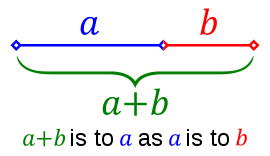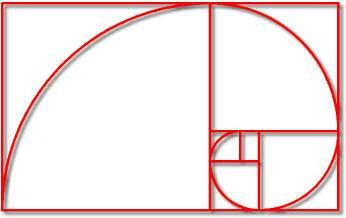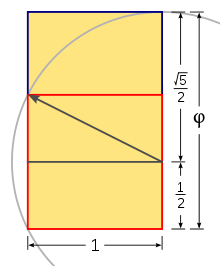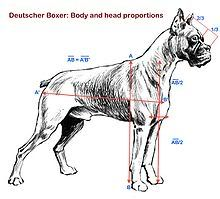###Author Topic: A new standard for Boxer muzzle length? (the golden ratio)  (Read 3410 times)

#### markwantsaboxer

• Fully Weaned
•• Posts: 88##### A new standard for Boxer muzzle length? (the golden ratio)
« on: May 07, 2010, 09:38:08 PM »
Perhaps you are familiar with the "golden ratio".From wikipedia:

"In mathematics and the arts, two quantities are in the golden ratio if the ratio of the sum of the quantities to the larger quantity is equal to (=) the ratio of the larger quantity to the smaller one. The golden ratio is an irrational mathematical constant, approximately 1.6180339887."In terms of percentages, in the golden ratio, line B is 38.1966011% the length of line A + B.

http://en.wikipedia.org/wiki/Golden_ratioFor a cool youtube video on this:As the video shows, the golden ratio is used widely in design; the computer screen you are looking into probably has a ratio of width over height that is the golden ratio (i.e., if it is ten inches high, it is probably 16.18 inches across).It is also found throughout nature. Famously, a nautilus shell expands in a spiral according to the proportions of the golden ratio.(This is known as a 'Fibonacci spiral' because it is also based on the Fibonacci sequence.)

This rate of expansion can be replicated graphically by taking a square (Square A) and then dividing it in half and then drawing a diagonal line across the subdivided half of Square A and using that diagonal as a radius to draw a circle; that circle can be used to expand the Square A into a rectangle.Now the long side of that rectangle can be used to make a big new square (Square B).

Now, let's get back to the Boxer muzzle.Again, in terms of percentages, in the golden ratio, line B is 38.1966011% the length of line A + B.

This can be the new Boxer breed standard for the ratio of muzzle length to total head length.  Intuitively, it would conform to the pleasing proportions of the golden ratio and it help would promote better temperature regulation in the breed because it would be a little bit longer than the current 33% ratio found in the standard.

We all have digital cameras and computers, right?So it's no problem nowadays to do this. If Frau Stockmann were around today, she'd have all her babies' mug shots on her netbook with a computer program estimating their very exact head and body proportions.Let's do it!"The muzzle: Muzzle length from the tip of the nose to the stop is 38.1966011% of total head length from the tip of the nose to the occiput."

#### BurningRiver##### Re: A new standard for Boxer muzzle length? (the golden ratio)
« Reply #1 on: May 22, 2010, 08:44:40 AM »
Except most breeders throughout the world today visually prefer a muzzle that is far shorter than the already proposed 1/3.Jessica, Mia and Carter
Burning River Boxers
http://www.burningriverboxers.com

In Heaven: Nedra, CGC 12/24/00-1/29/11